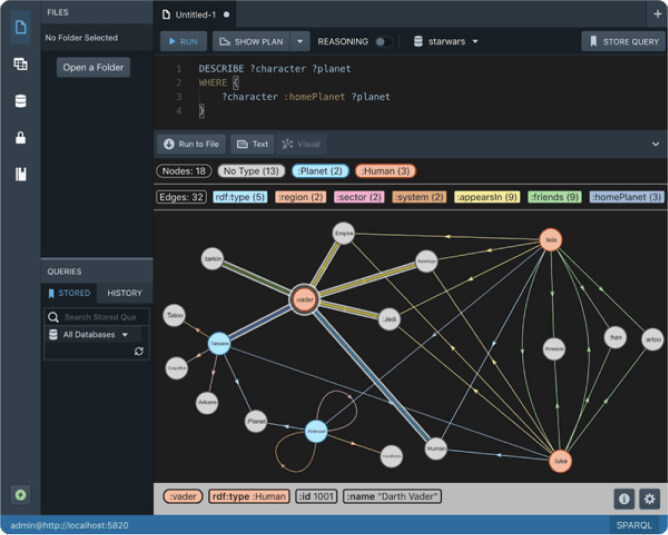Showing results for

Back to all articles

# Graph analytics with SparkStanislav Klenin Nov 17, 2021

Stardog Spark connector, coming out of beta with 7.8.0 release of Stardog, exposes data stored in Stardog as a Spark Dataframe and provides means to run standard graph algorithms from GraphFrames library. The beta version was sufficient for POC examples, but when confronted with real world datasets, its performance turned out not quite up to our standards for a GA release.

It quickly became obvious that the connector was not fully utilizing the distributed nature of Spark. How can it be improved?

## Partitioning in Datasource

The first idea involved partitioning at load time. Let’s take a look at this code snippet from the docs:

``````// Create a Stardog dataset
Dataset<Row> dataset = spark.read()
.format(StardogSource.class.getName())
.options(options)
``````

Behind the scenes, Spark constructs a Dataframe out of data retrieved from Stardog. `StardogSource`, implementation of Spark Datasource API, is responsible for the actual retrieval. This API defines several abstractions such as `Table` and `Scan`, and the `Partition` abstraction is of particular interest here. A Spark Datasource can opt for splitting underlying data into multiple partitions - so that the actual read operations can be performed in parallel.

For Stardog data source it means that a single query such as

``````CONSTRUCT { ?s ?p ?o } WHERE { ?s ?p ?o }
``````

becomes a series of queries with slices:

``````CONSTRUCT { ?s ?p ?o } WHERE { ?s ?p ?o } LIMIT N OFFSET K
``````

with fixed N and K varying between 0 and total data size divided by N. The total data size had to be specified as a separate configuration property, along with partition size. It can be calculated with a count query, and this would most likely be the preferred approach if results were encouraging… Unfortunately, they were not.

However, partitioning was still the right answer. It just had to be implemented differently.

## Partitioning Dataframes

Consider the following (simplified) example from GraphFrames Quickstart:

``````// Create a Vertex DataFrame with unique ID column "id"
val v = sqlContext.createDataFrame(List(
("a", "Alice", 34),
("b", "Bob", 36),
("c", "Charlie", 30)
)).toDF("id", "name", "age")

// Create an Edge DataFrame with "src" and "dst" columns
val e = sqlContext.createDataFrame(List(
("a", "b", "friend"),
("b", "c", "follow"),
("c", "b", "follow")
)).toDF("src", "dst", "relationship")

// Create a GraphFrame
val g = GraphFrame(v, e)
``````

GraphFrame is created from two Dataframes, and Stardog Spark connector does essentially the same thing. These two Dataframes, constructed from query results, can be partitioned using Spark APIs (either at Dataframe level or at the underlying RDD level).

After this, there was just one remaining catch. Dataframes API requires an “id” column for a vertex Dataframe. It can be any object uniquely identifying the vertex so one would expect the IRI returned from Stardog would work. And it does, somewhat. But performance of graph algorithms significantly degrades if this identifier is anything other than integer or long number. So the connector has to generate long ID for each vertex on the fly.

A new input parameter is introduced in 1.0 release of Spark connector to control this re-partitioning: `spark.dataset.repartition` (it defaults to 1 if not specified, meaning no re-partitioning).

## Benchmarks

To estimate performance impact of multiple partitions we used enwiki 2020 dataset. This dataset, originally in WebGraph format, has been converted to RDF database of the following structure:

``````:ID1 :links :ID2
``````

That is, vertices are IRIs constructed from string representation of integer IDs, and there is only one predicate, `:links`. This RDF database contains 140 million triples.

We ran PageRank algorithm with varying number of partitions, and the whole experiment was repeated for different Spark cluster configurations.

One common thing in these experiment is the time needed to retrieve data from Stardog instance to the Spark cluster. For this dataset it is 6 minutes, and it is included in the results listed below.

Results for Spark cluster with 10 m5.2xlarge workers (plus 1 master):With smaller number of partitions there is a possibility of Spark cluster nodes running out of memory, and this is the case here with 10 partitions. Performance improves as the number of partitions increases, but it saturates after some point (around two times number of worker nodes for this dataset).

Another way to improve performance is to restrict the dataset - either explicitly include only vertices you need, or filter out elements you don’t need. This is why the connector supports custom queries for graph analytics via `stardog.query` configuration property.

Here is an example query to build a graph without any literals. That is, only IRI to IRI edges are included while IRI to literal edges such as `ProductType1 rdfs:label "Thing"` are ignored:

``````stardog.query=CONSTRUCT { ?s ?p ?o } WHERE { ?s ?p ?o FILTER(!isLITERAL(?o)) }
``````

For the Wikipedia dataset mentioned above it is meaningless since all vertices are IRIs. However, with other datasets this filter can make significant difference. This is the difference for the BSBM dataset of 100 million triples, using 10 m5.2xlarge workers with 20 partitions:It is worth noting that this approach was applicable to the beta version as well. In the GA release with re-partitioning it can still help, but better use of Spark parallelism means it may be needed less frequently.

This concludes the road from beta to the GA release for Stardog Graph Analytics. While there is still room for improvement, the connector is ready for real use cases. You can experiment with it using your own Spark cluster and data - follow the steps in the docs and let us know how it went in Stardog Community!